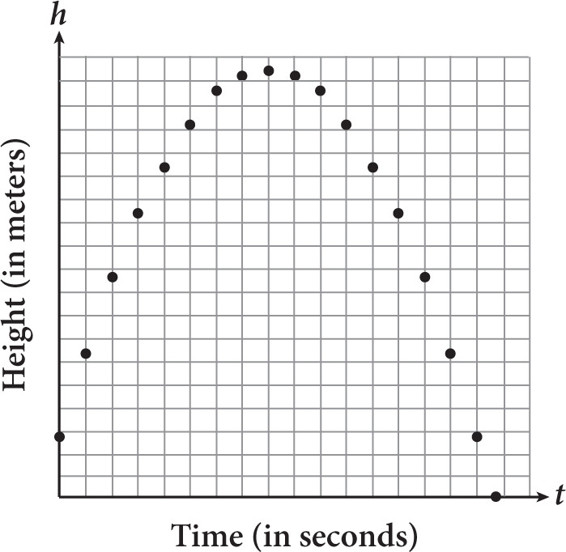# SAT Math Multiple Choice Question 273: Answer and Explanation

### Test Information

Question: 273

3.A physics class is using simulation software to study water bottle rockets before attempting to build one for the National Physics Competition. Their first simulation is of a rocket without a parachute launched from the roof of the gymnasium. The scatterplot shows the approximate path of the rocket. The software program generates the equation h = –4.9t2 + 39.2t + 14 to model the data, where h is the height in meters of the rocket t seconds after it was launched. What does the number 14 most likely represent in this equation?

• A. The number of seconds the rocket was in the air
• B. The height of the gymnasium from which the rocket was launched
• C. The number of seconds that it took the rocket to reach its maximum height
• D. The maximum height of the rocket